Courses

# Absorption Accompanied By Irreversible Reactions Chemical Engineering Notes | EduRev

## Mass Transfer

Created by: Telecom Tuners

## Chemical Engineering : Absorption Accompanied By Irreversible Reactions Chemical Engineering Notes | EduRev

The document Absorption Accompanied By Irreversible Reactions Chemical Engineering Notes | EduRev is a part of the Chemical Engineering Course Mass Transfer.
All you need of Chemical Engineering at this link: Chemical Engineering

4.9.3. Absorption accompanied by irreversible mth order reactions:
According to film model when a gas component is subject to an mth or pseudomth order reaction, the absorption rate is expressed by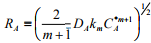(4.60)
By introducing M for the mth-order reaction, the absorption rate can be expressed as: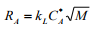(4.61)
where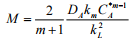(4.62)
As an example, the oxygen takes part in a second-order reaction in the sulphite oxidation system which is often used for comparing gas-liquid interfacial area in various reactors. A more detailed information of mth order reactions can be found from article published by Hikita and Asai (1964).

4.10.4. Absorption accompanied by irreversible second order reactions:
The gas-liquid reactions are found in many chemical industries where a gas component A reacts with a liquid component B as: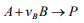(4.63)
The rate of absorption depends on the reaction range of absorption. In Table 4.1, the various formulas for absorption rate at different reaction range are given (Deckwer, 1992).

Table 4.1: Formula for calculating absorption rate during second-order-reaction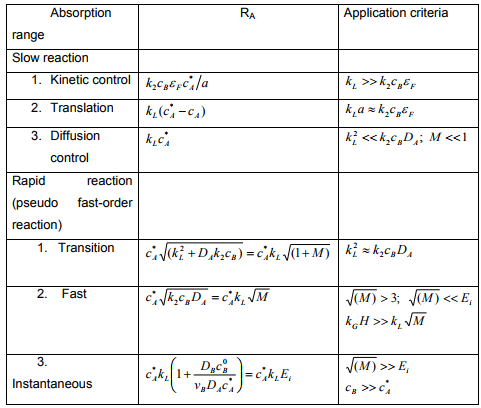Various situations at the interface during reactions:
Case (a): The liquid component B is not significantly broken at the interface if the concentration of B is large in comparison with cA, then it reduces to a pseudofirst-order reaction.
Case (b): When components A and B react so quickly that they cannot coexist at the same location to any significant extent (‘instantaneous reaction’). The film model gives for this case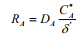(4.64)
where  δ' is the reaction plane at the condition vBjA = -jin which the concentration of both components is equal to zero. It is a function of the diffusion rate of A and B and of the whole boundary thickness which can be expressed as: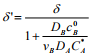(4.65)
Substituting the Equation (4.65) in Equation (4.64), the absorption rate can be written as: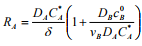(4.66)
which follows since kL = DA/δ: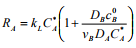(4.67)
The expression in brackets called the enhancement factor Ei due to instantaneous reaction.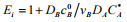(4.68)
From Equation (4.67), if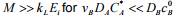then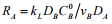(4.69)
The absorption rate is entirely controlled by the diffusion of B and is independent of C*and partial pressure pA.

Case (c): If the concentration of B drops distinctly in comparison with the bulk concentration, yet does not reach zero, the film model produces two coupled differential equations which can be solved numerically. Van Krevelen and Hoftijzer (1948) have provided an approximated solution as: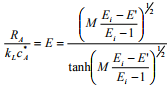(4.70)
where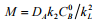(4.71)
Ei is given by Equation (4.68). From the Equation (4.70) it is observed that for various Ei, E increases for a given Ei with increasing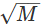as long as E =Ei. Hence the expression for Ei can be used for E, if>10Ei and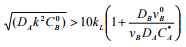(4.72)
On the other hand E is near the diagonal E =and subsequent products are based on a pseudo-first-order reaction if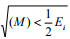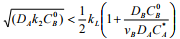(4.73)
The van Krevelen and Hoftijzer Equation (4.70) does not give an explicit value for E (Deckwer, 1992). Wellek et al. (1978) provided further details and recommended the following explicit equation for the calculation of E: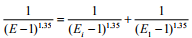(4.74)

where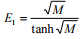(4.75)

Offer running on EduRev: Apply code STAYHOME200 to get INR 200 off on our premium plan EduRev Infinity!

,

,

,

,

,

,

,

,

,

,

,

,

,

,

,

,

,

,

,

,

,

;